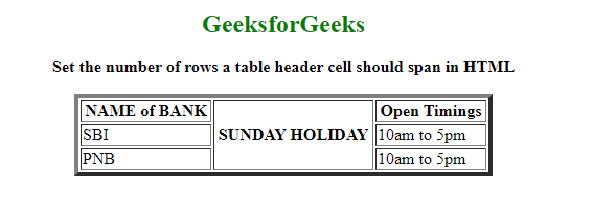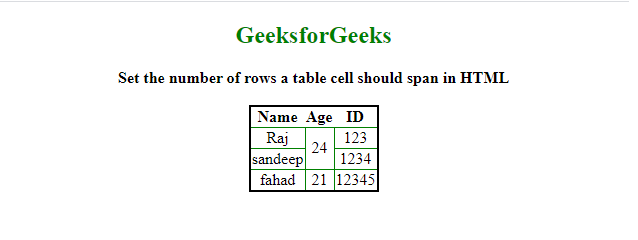Open In App
Related Articles
• Write an Interview Experience

# How to set the number of rows a table cell should span in HTML ?

In this article, we will show how multiple rows are spanned by a table header cell. The task can be done by using the rowspan attribute while using the <th> tag. This property is used to merge one or more cells as well as increase the height of the single header cell automatically.

Syntax:

`<th rowspan = "value">table content...</th>`

Example: In this example, we will set the number of rows a table header cell should span in HTML

## HTML

 ```<``html``>` `<``body``>``    ``<``center``>``        ``<``h2` `style``=``"color: green"``>GeeksforGeeks``        ``<``b``>``            ``Set the number of rows a table header``            ``cell should span in HTML``        ````        ``<``table` `border``=``"4"` `width``=``"400"``>``            ``<``tr``>``                ``<``th``>NAME of BANK``                ``<``th` `rowspan``=``"3"``>SUNDAY HOLIDAY``                ``<``th``>Open Timings``            ````            ``<``tr``>``                ``<``td``>SBI``                ``<``td``>10am to 5pm``            ````            ``<``tr``>``                ``<``td``>PNB``                ``<``td``>10am to 5pm``            ````        ````    ````` ``

Output:Example 2: In this example. we will learn, how rows can be made to span over multiple cells.

The task can be done by using the rowspan attribute when using the <td> tag within <tr> tag.  It allows the single table cell to span the height of more than one cell. It contains a numeric value that defines the number of rows that should be spanned.

Syntax:

```<tr>
<td rowspan = "value">table column content...</td>
</tr>```

## HTML

 ```<``html``>` `<``head``>``    ``<``style``>``        ``table {``            ``border: 2px solid black;``            ``border-collapse: collapse;``            ``padding: 6px;``        ``}``        ``td {``            ``border: 1px solid green;``            ``text-align: center;``        ``}``    ````` `<``body` `style``=``"text-align: center"``>``    ``<``center``>``        ``<``h2` `style``=``"color: green"``>GeeksforGeeks``        ``<``b``>``            ``Set the number of rows a table``            ``cell should span in HTML``        ````        ``<``br` `/>``        ``<``table``>``            ``<``tr``>``                ``<``th``>Name``                ``<``th``>Age``                ``<``th``>ID``            ````            ``<``tr``>``                ``<``td``>Raj``                ````                ``<``td` `rowspan``=``"2"``>24``                ``<``td``>123``            ````            ``<``tr``>``                ``<``td``>sandeep``                ``<``td``>1234``            ````            ``<``tr``>``                ``<``td``>fahad``                ``<``td``>21``                ``<``td``>12345``            ````        ````    ````` ``

Output: